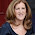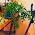### MATH 1280 Introduction to Statistics – Assignment 2 – Part 3

Gardenia for illustration
Part 3 - Answer for question 9 to 15

Assuming that you read the flowers.csv file into an R object called flower.data, run the following R code (do not paste the ">” character into R). Note that we are not rounding the numbers here. Use the output for the next five tasks:”
> table(flower.data\$Sepal.Width)
> plot(table(flower.data\$Sepal.Width))

I changed the code to be (the result is the same):
> table(fl.1\$Sepal.Width)
> plot(table(fl.1\$Sepal.Width))

Results:
2 2.2 2.3 2.4 2.5 2.6 2.7 2.8 2.9 3 3.1 3.2 3.3 3.4 3.5 3.6 3.7 3.8 3.9 4 4.1 4.2 4.4
1 3 4 3 8 5 9 14 10 26 11 13 6 12 6 4 3 6 2 1 1 1 1

Graph

Question 9. “What is the sum of the first three frequencies in the frequency table for sepal width?”
Answer:  The sum of the first three frequencies is 8

Question 10. “What does your answer to the previous question represent (in terms of sepal width and frequency and the percentage of all sepal measurements)”
It is shown that there are 8 flowers with “Sepal width” less than and equal to 2.3. Or, in other words, we may say that about 5.33% of the flowers’ “Sepal width” are less than and equal to 2.3.

One of Picasso paintings for illustration only

Question 11. “What is the sum of the last three frequencies in the frequency table for sepal width.”
Answer: The sum of the last three frequencies is 3

Question 12. “How many flowers in the sample had sepal widths less than 4 (do NOT round the sepal width numbers for this, but you can round your final answer to 3 decimal places)?”

Picasso painting, illustration only

Question13. “What does the tallest bar in the plot represent?”
Answer: It represents the most occurring of “sepal with” of flowers. We found that 3 is the most occurring of “Sepal width”

Question 14. “Create a frequency table that shows the frequencies for each species of flower in the sample. Paste your R command and output into your answer (do NOT display data from a data frame, display data using the table() command):”
Result:
setosa versicolor virginica
50 50 50

Or: > table(fl.1\$Species)
Result:
setosa versicolor virginica
50 50 50

Illustration of Picasso painting

Question 15. “Explain two things about the table that you created for the previous task:”
*Why did the frequency table for flower species contain words in the first row as opposed to numbers?
Answer: The reason is simple that “flower species” is variable contains factor type data, not numeric type data. Thus, the first row contains words (factor) as opposed to numbers (numeric).

**What is the meaning of the numbers in the second row of the table?

Answer: Since the number in the second row represent the frequency, thus it means that how many times which species occurs in the flower.data. In this case, there are 50 sentosas, 50 versicolors and 50 virginicas species.

1.Yes, I have frozen fresh strawberries, and I'd say they'd last maybe a month; perhaps a little longer.

1.Thank you to visit my article:
MATH 1280 Introduction to Statistics – Assignment 2 – Part 3
@
Gardenia for illustration
Part 3 - Answer for question 9 to 15

“Assuming that you read the flowers.csv file into an R object called flower.data, run the following R code (do not paste the ">” character into R). Note that we are not rounding the numbers here. Use the output for the next five tasks:”
> table(flower.data\$Sepal.Width)
> plot(table(flower.data\$Sepal.Width))

I changed the code to be (the result is the same):

> table(fl.1\$Sepal.Width)

> plot(table(fl.1\$Sepal.Width))

Results:

2 2.2 2.3 2.4 2.5 2.6 2.7 2.8 2.9 3 3.1 3.2 3.3 3.4 3.5 3.6 3.7 3.8 3.9 4 4.1 4.2 4.4

1 3 4 3 8 5 9 14 10 26 11 13 6 12 6 4 3 6 2 1 1 1 1

2.MATH 1280 Introduction to Statistics – Assignment 2 – Part 3
@
Question 15. “Explain two things about the table that you created for the previous task:”

*Why did the frequency table for flower species contain words in the first row as opposed to numbers?

Answer: The reason is simple that “flower species” is variable contains factor type data, not numeric type data. Thus, the first row contains words (factor) as opposed to numbers (numeric).

**What is the meaning of the numbers in the second row of the table?

Answer: Since the number in the second row represent the frequency, thus it means that how many times which species occurs in the flower.data. In this case, there are 50 sentosas, 50 versicolors and 50 virginicas species.

3.MATH 1280 Introduction to Statistics – Assignment 2 – Part 3
-

“Assuming that you read the flowers.csv file into an R object called flower.data, run the following R code (do not paste the ">” character into R). Note that we are not rounding the numbers here. Use the output for the next five tasks:”
> table(flower.data\$Sepal.Width)
> plot(table(flower.data\$Sepal.Width))

I changed the code to be (the result is the same):

> table(fl.1\$Sepal.Width)

> plot(table(fl.1\$Sepal.Width))

Results:

2 2.2 2.3 2.4 2.5 2.6 2.7 2.8 2.9 3 3.1 3.2 3.3 3.4 3.5 3.6 3.7 3.8 3.9 4 4.1 4.2 4.4

1 3 4 3 8 5 9 14 10 26 11 13 6 12 6 4 3 6 2 1 1 1 1

2.Waaaah... ini memusingkan, tapi semoga artikel ini bermanfaat untuk teman-teman yang membutuhkan.
Karena aku bukan anak matematika... jadi, aku butuh waktu lama untuk memahaminya. :D Hehe...

Semangat dan sukses Kak.

1.Ralat:

Suami: semua

2.Thank you to visit my article:
MATH 1280 Introduction to Statistics – Assignment 2 – Part 3
@
Question 9. “What is the sum of the first three frequencies in the frequency table for sepal width?”

Answer: The sum of the first three frequencies is 8

3.MATH 1280 Introduction to Statistics – Assignment 2 – Part 3
-
“Assuming that you read the flowers.csv file into an R object called flower.data, run the following R code (do not paste the ">” character into R). Note that we are not rounding the numbers here. Use the output for the next five tasks:”
> table(flower.data\$Sepal.Width)
> plot(table(flower.data\$Sepal.Width))

I changed the code to be (the result is the same):
> table(fl.1\$Sepal.Width)
> plot(table(fl.1\$Sepal.Width))

Results:
2 2.2 2.3 2.4 2.5 2.6 2.7 2.8 2.9 3 3.1 3.2 3.3 3.4 3.5 3.6 3.7 3.8 3.9 4 4.1 4.2 4.4

1 3 4 3 8 5 9 14 10 26 11 13 6 12 6 4 3 6 2 1 1 1 1

4.MATH 1280 Introduction to Statistics – Assignment 2 – Part 3
-
Question 9. “What is the sum of the first three frequencies in the frequency table for sepal width?”

Answer: The sum of the first three frequencies is 8

3.Wah matematika saya terlalu rendah nih.

1.Thank you to visit my article:
MATH 1280 Introduction to Statistics – Assignment 2 – Part 3
@
Question 10. “What does your answer to the previous question represent (in terms of sepal width and frequency and the percentage of all sepal measurements)”

It is shown that there are 8 flowers with “Sepal width” less than and equal to 2.3. Or, in other words, we may say that about 5.33% of the flowers’ “Sepal width” are less than and equal to 2.3.

2.MATH 1280 Introduction to Statistics – Assignment 2 – Part 3
-
Question 11. “What is the sum of the last three frequencies in the frequency table for sepal width.”

Answer: The sum of the last three frequencies is 3

4.1.Thank you to visit my article:
MATH 1280 Introduction to Statistics – Assignment 2 – Part 3
@
Question 11. “What is the sum of the last three frequencies in the frequency table for sepal width.”

Answer: The sum of the last three frequencies is 3

2.MATH 1280 Introduction to Statistics – Assignment 2 – Part 3
-
Question 12. “How many flowers in the sample had sepal widths less than 4 (do NOT round the sepal width numbers for this, but you can round your final answer to 3 decimal places)?”

3.MATH 1280 Introduction to Statistics – Assignment 2 – Part 3

5.Too complicated for me!

1.Thank you to visit my article:
MATH 1280 Introduction to Statistics – Assignment 2 – Part 3
@
Question 12. “How many flowers in the sample had sepal widths less than 4 (do NOT round the sepal width numbers for this, but you can round your final answer to 3 decimal places)?”

2.MATH 1280 Introduction to Statistics – Assignment 2 – Part 3
-
Question13. “What does the tallest bar in the plot represent?”

Answer: It represents the most occurring of “sepal with” of flowers. We found that 3 is the most occurring of “Sepal width”

6.interesting information ☺
greetings
Lili

1.Thank you to visit my article:
MATH 1280 Introduction to Statistics – Assignment 2 – Part 3
@
Question13. “What does the tallest bar in the plot represent?”

Answer: It represents the most occurring of “sepal with” of flowers. We found that 3 is the most occurring of “Sepal width”

2.MATH 1280 Introduction to Statistics – Assignment 2 – Part 3
@
Question 14. “Create a frequency table that shows the frequencies for each species of flower in the sample. Paste your R command and output into your answer (do NOT display data from a data frame, display data using the table() command):”

Result:

setosa versicolor virginica

50 50 50

Or: > table(fl.1\$Species)

Result:

setosa versicolor virginica

50 50 50

3.MATH 1280 Introduction to Statistics – Assignment 2 – Part 3

7.A lot of thanks for sharing your personal experience. I just learned a lot.
Photo Editing
clipping path service

1.Thank you to visit my article:
MATH 1280 Introduction to Statistics – Assignment 2 – Part 3
@
Question 14. “Create a frequency table that shows the frequencies for each species of flower in the sample. Paste your R command and output into your answer (do NOT display data from a data frame, display data using the table() command):”

Result:

setosa versicolor virginica

50 50 50

Or: > table(fl.1\$Species)

Result:

setosa versicolor virginica

50 50 50

2.MATH 1280 Introduction to Statistics – Assignment 2 – Part 3
@
Question 15. “Explain two things about the table that you created for the previous task:”

*Why did the frequency table for flower species contain words in the first row as opposed to numbers?

Answer: The reason is simple that “flower species” is variable contains factor type data, not numeric type data. Thus, the first row contains words (factor) as opposed to numbers (numeric).

**What is the meaning of the numbers in the second row of the table?

Answer: Since the number in the second row represent the frequency, thus it means that how many times which species occurs in the flower.data. In this case, there are 50 sentosas, 50 versicolors and 50 virginicas species.

3.MATH 1280 Introduction to Statistics – Assignment 2 – Part 3